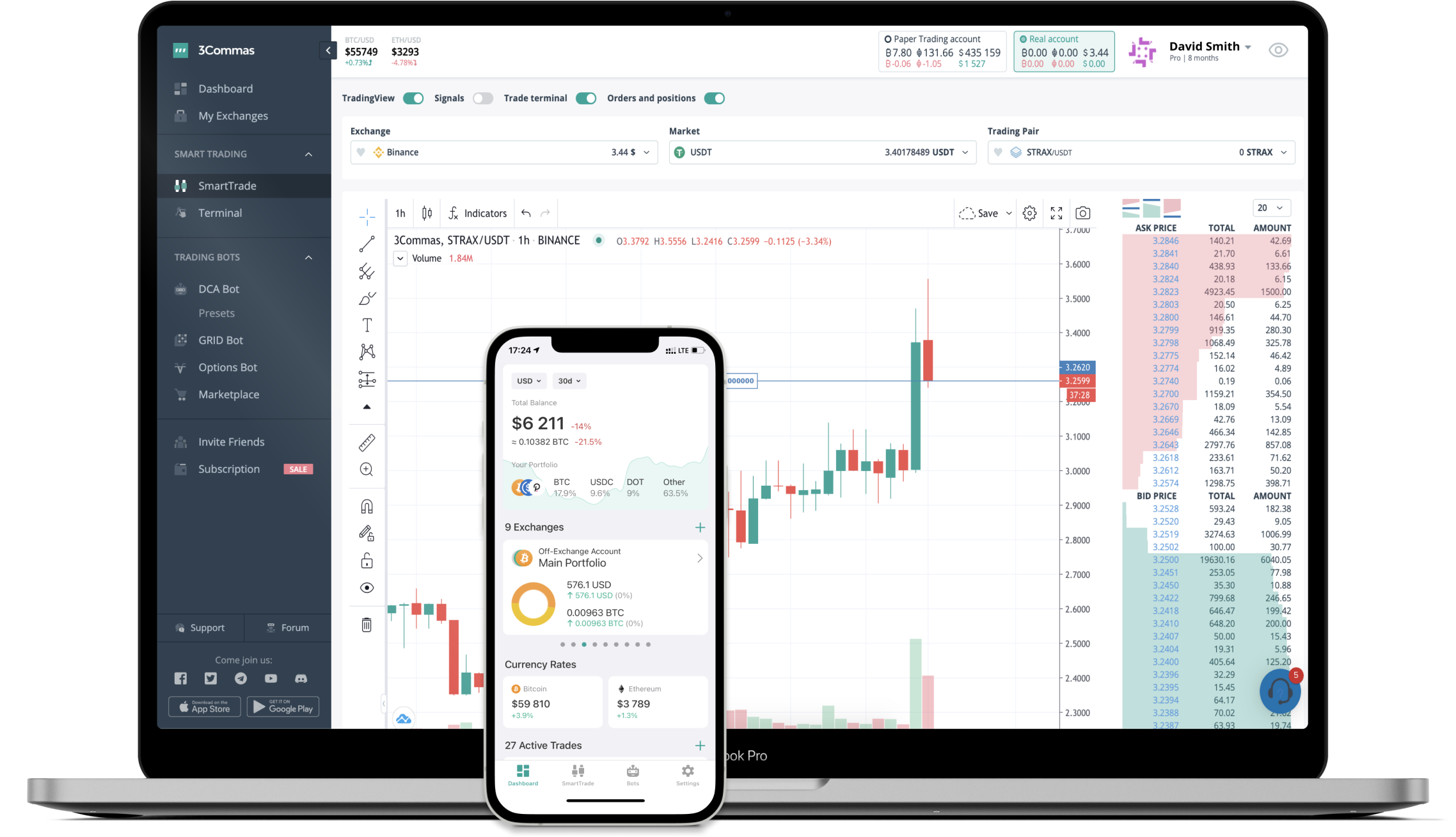# Convert 10 DIVI to ETH (Divi to Ethereum)

Divi
PriceETH 0.00000693-9.13%
VolumeETH 75
How it work

The 3Commas currency calculator allows you to convert a currency from Divi (DIVI) to Ethereum (ETH) in just a few clicks at live exchange rates.

Simply enter the amount of Divi you wish to convert to ETH and the conversion amount automatically populates. You can also use our Prices Calculator Table to calculate how much your currency is worth in other denominations, i.e. .1 DIVI, .5 DIVI, 1 DIVI, 5 DIVI, or even 10 DIVI.

## DIVI to ETH Exchange Calculator

The chart displays Divi price changes in ETH.

The ultimate tools for crypto traders to maximize trading profits while minimizing risk and loss.## Latest DIVI to ETH price calculator

Divi market price is updated every three minutes and is automatically displayed in ETH. Below are the most popular denominations to convert to ETH.

Divi
Ethereum
0.00
ETH
0.00
ETH
0.00
ETH
0.00
ETH
0.00
ETH
0.00
ETH
0.00
ETH
0.00
ETH
0.00
ETH
0.00
ETH
0.00
ETH
0.00
ETH
0.01
ETH
0.02
ETH
Ethereum
Divi
1443.00144300
DIVI
14430.01443001
DIVI
144300.14430014
DIVI
288600.28860029
DIVI
432900.43290043
DIVI
721500.72150072
DIVI
1443001.44300144
DIVI
3607503.60750361
DIVI
7215007.21500722
DIVI
14430014.43001443
DIVI
36075036.07503608
DIVI
72150072.15007215
DIVI
144300144.30014430
DIVI
360750360.75036075
DIVI

## FAQ

### How much is 1 Divi in Ethereum?

Divi price in ETH is constantly changing.

At this moment, 1 Divi equals 0.00000693 ETH

### How do I calculate the conversion from DIVI to ETH?

The 3Commas Divi Calculator allows you to easily calculate the conversion price of DIVI to ETH by simply entering the amount of Divi in the corresponding field and will automatically convert the value in Ethereum (ETH).

You can also use our Divi price table above to check the latest Divi price in major fiat and crypto currencies.

### How can I convert Divi to ETH?

The most common way of converting DIVI to ETH is by using a Crypto Exchange or a P2P (person-to-person) exchange platform like LocalBitcoins, etc.